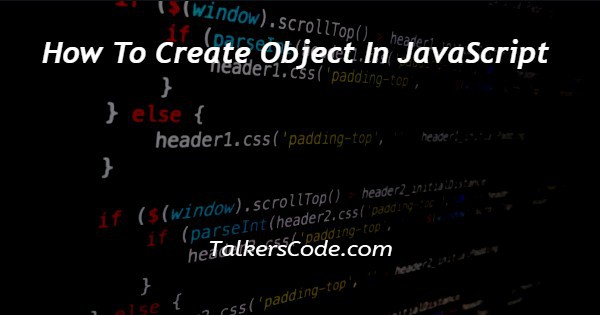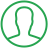# How To Create Object In JavaScript

Last Updated : Jul 1, 2023In this tutorial we will show you the solution of how to create object in JavaScript, there are many ways with help of which we can create objects in JavaScript.

Let us see the example below to understand this concept with help of codes.

## Step By Step Guide On How To Create Object In JavaScript :-

There are many ways with help of we can create an object using JavaScript. The ways are:

• With the help of the object’s literal
• With the help of a constructor
• With the help of an object.create()

In this example, we are going to understand this article with the help of our first way which is using an object’s literal.

Below, we will show you an example with the help of codes. Let us understand the example given for quick learning.

```// using the object’s literal
<script>
let details = {
};
console.log(details.email);
console.log(details ['email']);
</script>```
1. As we here see that we today write only JavaScript codes in our example.
2. In previous tutorials, we already many times create a full code example in which we first create an HTML form with its basic structure. We use HTML, head, title, body, and script tags inside that structure.
3. In this example, as we see only, we write our JavaScript codes. The paragraph tag used here is only for display outputs or we can also use console instead of HTML tags. Now, let us understand our examples one by one.
4. And in these codes you see that we create an object. And in this object literal example, which is given above. In this example we create object in key value pair.
5. We can also create a simple object like one dimensional array. One thing to remember here that when we are going to create an object using object literal when have to use curly braces that are {}, and after that we just given the name to this object by use const.
6. Now, to see that the object is create successfully. We have to access the data of object. So, for this we use console.
7. You can also use another way to display the data, but we use console here. Now, at last thing we can access the data in two ways. The way which we use most is first one. In which we have to use period(.).
8. Whereas in another case we access the object data like array, in which we use key name with square braces. You can see the above example for reference.

## Conclusion :-

At last, in conclusion, here we can say that with the help of this article we can understand how to create objects using JavaScript.

I hope this tutorial on how to create object in JavaScript helps you and the steps and method mentioned above are easy to follow and implement.

##About Dikshita

Passionate Electronics and Communication Engineering student with expertise in web development (HTML, CSS, JS,PHP, Bootstrap, React.js) and content writing. Eager problem solver and tech enthusiast, adept at creating engaging web experiences.